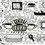# Reversing Digits

Let $$N_{1}=\overline{abcd}$$ and $$N_{2}=\overline{dcba}$$ be two $$4$$ digits positive integers.

Let $e$ be a positive integer such that $N_{1} \times e$ = $N_{2}$.

How many ordered sets of integers $a,b,c,d$ and $e$ are there?

It's not necessary that $a,b,c,d$ are distinct.

$A$ $request$ : Please explain your steps a bit if you use Modular Arithmetic because I ain't much used to it.Note by Abhimanyu Swami
6 years, 11 months ago

This discussion board is a place to discuss our Daily Challenges and the math and science related to those challenges. Explanations are more than just a solution — they should explain the steps and thinking strategies that you used to obtain the solution. Comments should further the discussion of math and science.

When posting on Brilliant:

• Use the emojis to react to an explanation, whether you're congratulating a job well done , or just really confused .
• Ask specific questions about the challenge or the steps in somebody's explanation. Well-posed questions can add a lot to the discussion, but posting "I don't understand!" doesn't help anyone.
• Try to contribute something new to the discussion, whether it is an extension, generalization or other idea related to the challenge.

MarkdownAppears as
*italics* or _italics_ italics
**bold** or __bold__ bold
- bulleted- list
• bulleted
• list
1. numbered2. list
1. numbered
2. list
Note: you must add a full line of space before and after lists for them to show up correctly
paragraph 1paragraph 2

paragraph 1

paragraph 2

[example link](https://brilliant.org)example link
> This is a quote
This is a quote
    # I indented these lines
# 4 spaces, and now they show
# up as a code block.

print "hello world"
# I indented these lines
# 4 spaces, and now they show
# up as a code block.

print "hello world"
MathAppears as
Remember to wrap math in $$ ... $$ or $ ... $ to ensure proper formatting.
2 \times 3 $2 \times 3$
2^{34} $2^{34}$
a_{i-1} $a_{i-1}$
\frac{2}{3} $\frac{2}{3}$
\sqrt{2} $\sqrt{2}$
\sum_{i=1}^3 $\sum_{i=1}^3$
\sin \theta $\sin \theta$
\boxed{123} $\boxed{123}$

Sort by:

Case-by-case analysis is not too bad. Consider $e=1$. Then from the original statement we know that $a=d, b=c$, which gives us $9\cdot 10=90$ sets.

Suppose now $e>1$. For the rest of the solution we have $(a+1)e>d>a$.

We then check for sets $(a,d,e)$ that satisfy the above inequality and $a$ is the last digit of $de$. Note that we just need to check for $a<\frac{10}{e}$, which is not a lot, that is if we fix $a$ and $e$, there are only $12$ valid pairs of $(a, e)$ just by $1. One gets $(a,d,e)=(2,8,4), (1,9,9)$.

Case 1: $(a,d,e)=(2,8,4)$

From the original statement, we arrive at $400b+40c+30=100c+10b$ which simplifies to $390b+30=60c$. Therefore $b<2$. Case-by-case analysis for $b=(0,1)$ gives us only $(b,c)=(1,7)$, which gives us the ordered set $(a,b,c,d,e)=(2,1,7,8,4)$.

Case 2: $(a,d,e)=(1,9,9)$

Similarly, we have $900b+90c+80=100c+10b$ which simplifies to $890b+80=10c$. Therefore $b=0$, which gives $c=8$, which gives us the ordered set $(a,b,c,d,e)=(1,0,8,9,9)$.

We then conclude there are 92 such sets.

- 6 years, 11 months ago

Can you explain, why is $(a+1)e>d$, By the way, nice solution. Now can you please solve this one?

- 6 years, 11 months ago

Suppose $d>ae+e$. Then $1000e\leq e\cdot \overline{bcd}\leq 999e$, which is a contradiction.

- 6 years, 11 months ago

You can prove it by setting e equal to 1 or e larger than 1 so there are less steps involved

- 6 years, 11 months ago

Since $1234*9$ is more than $10000$ we find that e is less than 9. A case by case analysis might work here.

- 6 years, 11 months ago

But won't that be too long? And remember I didn't say that $a,b,c,d$ are distinct. They may or may not be.

- 6 years, 11 months ago